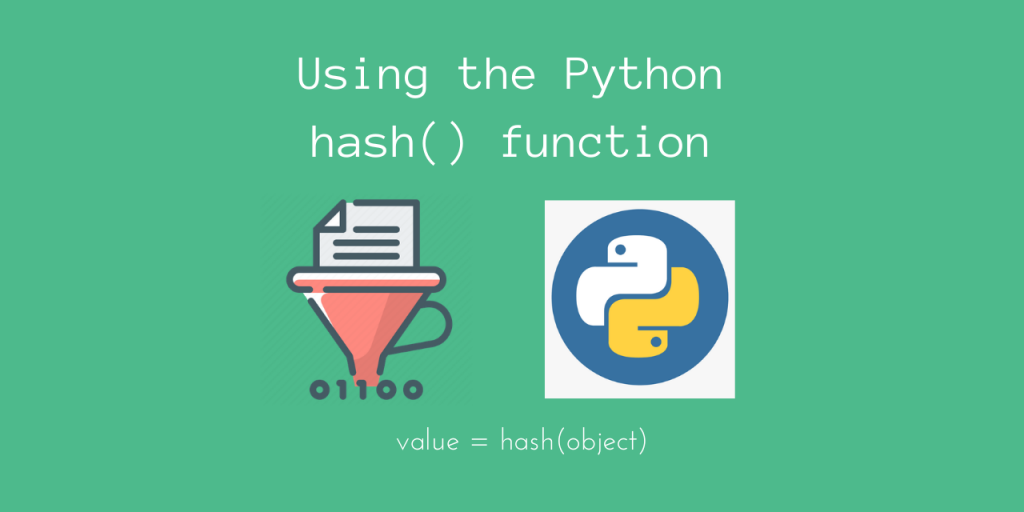# Using the Python hash() functionHello everyone! In today’s article, we’ll be looking at Python’s in-built `hash()` function. The Python `hash()` function computes the hash value of a Python object. But the language uses this to a large extent.

## Basic Syntax of Python hash()

This function takes in an immutable Python object, and returns the hash value of this object.

```value = hash(object)
```

Remember that the hash value is dependent on a hash function, (from `__hash__()`), which `hash()` internally calls. This hash function needs to be good enough such that it gives an almost random distribution.

Well, why do we want a hash function to randomize its values to such a large extent? This is because we want the hash function to map almost every key to a unique value.

If your values are randomly distributed, there will be very little chance of two different keys being mapped to the same value, which is what we want!

Now, let’s look at the `hash()` function in use, for simple objects like integers, floats and strings.

## Using the hash() function – Some Examples

```int_hash = hash(1020)

float_hash = hash(100.523)

print(f"For {1020}, Hash : {int_hash}")
print(f"For {100.523}, Hash: {float_hash}")
print(f"For {'Hello from AskPython'}, Hash: {string_hash}")
```

Output

```For 1020, Hash : 1020
For 100.523, Hash: 1205955893818753124
For Hello from AskPython, Hash: 5997973717644023107
```

As you can observe, integers have the same hash value as their original value. But the values are obviously different for the float and the string objects.

Now, it won’t be very safe if the same object (except integers/floats) always has the same hash value. So, if you run the above snippet again, you’ll notice different values!

For example, this is my output when I run the same snippet for the second time.

```For 1020, Hash : 1020
For 100.523, Hash: 1205955893818753124
For Hello from AskPython, Hash: -7934882731642689997
```

As you can see, the value is changed for the string! This is a good thing because it prevents the same object from being potentially accessed by someone! The hash value remains constant only until the duration of your program.

After that, it keeps changing every time you run your program again.

## Why cannot we use hash() on mutable objects?

Now, remember that we mentioned earlier about `hash()` being used only on immutable objects. What does this mean?

This means that we cannot use `hash()` on mutable objects like lists, sets, dictionaries, etc.

```print(hash([1, 2, 3]))
```

Output

```TypeError: unhashable type: 'list'
```

Why is this happening? Well, it would be troublesome for the program to keep changing the hash value every time the value of a mutable object changes.

This will make it very time consuming to keep updating the hash value again. If you do this, then Python needs to take a lot of time to keep referring to the same object, since the references will keep changing!

Due to this, we cannot hash mutable objects using `hash()`, since they only have a single value, which is hidden from us, so that the program can internally keep a reference to it.

However, we can use `hash()` on an immutable tuple. This is a tuple that consists of only immutable objects, like ints, floats, etc.

```>>> print(hash((1, 2, 3)))
2528502973977326415

>>> print(hash((1, 2, 3, "Hello")))
-4023403385585390982

>>> print(hash((1, 2, [1, 2])))
Traceback (most recent call last):
File "<stdin>", line 1, in <module>
TypeError: unhashable type: 'list'
```

## Using hash() on a Custom Object

Since the default Python `hash()` implementation works by overriding the `__hash__()` method, we can create our own `hash()` method for our custom objects, by overriding `__hash__()`, provided that the relevant attributes are immutable.

Let’s create a class `Student` now.

We’ll be overriding the `__hash__()` method to call `hash()` on the relevant attributes. We will also be implementing the `__eq__()` method, for checking equality between the two custom objects.

```class Student:
def __init__(self, name, id):
self.name = name
self.id = id

def __eq__(self, other):
# Equality Comparison between two objects
return self.name == other.name and self.id == other.id

def __hash__(self):
# hash(custom_object)
return hash((self.name, self.id))

student = Student('Amit', 12)
print("The hash is: %d" % hash(student))

# We'll check if two objects with the same attribute values have the same hash
student_copy = Student('Amit', 12)
print("The hash is: %d" % hash(student_copy))
```

Output

```The hash is: 154630157590
The hash is: 154630157597
```

We can indeed observe the hash of our custom object. Not only that; two different objects even with the same attribute values, have different hash values!

This is indeed what we want to expect from a hash function, and `hash()` has successfully given us that!

## Conclusion

We learned about using the Python `hash()` function. This is very useful for the program to maintain references to each object, using a special integer value.

We also saw how we could make `hash()` work on custom objects, provided it’s attributes are immutable.

## References

• JournalDev article on Python hash() function